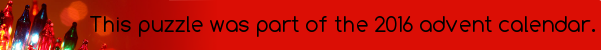mscroggs.co.uk
mscroggs.co.uksubscribe

# Puzzles

## 5 DecemberToday's number is the number of ways that 35 can be written as the sum of distinct numbers, with none of the numbers in the sum being divisible by 9.
Clarification: By "numbers", I mean (strictly) positive integers. The sum of the same numbers in a different order is counted as the same sum: eg. 1+34 and 34+1 are not different sums. The trivial sum consisting of just the number 35 counts as a sum.

## Archive

Show me a random puzzle
▼ show ▼
© Matthew Scroggs 2012–2021